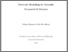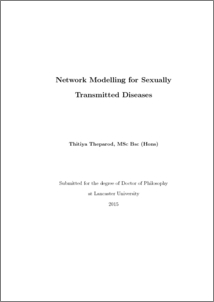Network modelling for sexually transmitted diseases

Theparod, Thitiya (2015) Network modelling for sexually transmitted diseases. PhD thesis, UNSPECIFIED.Preview
PDF (2015ThitiyaPhD)
2015ThitiyaPhD.pdf - Published Version

Abstract

The aim of this thesis is to propose suitable mathematical models for the analysis of sexually transmitted disease epidemics. We are interested in a closed population, where infections are only transmitted through heterosexual contacts. The population is hence divided into two groups: male and female. Individuals are classified according to gender, relationship and disease status. Both stochastic and deterministic SIS models are employed. The stochastic models are formulated in terms of a Markov process with a finite state space. Two main models are constructed and quantities of interest such as the basic reproduction number and endemic level of the sexually transmitted disease (STD) are obtained. The first model is formulated to describe dynamics of STDs, where the sexual behaviour is considered “faithful”. By being faithful, we mean individuals are monogamous, and there are no casual sexual contacts (one-night stands). The early stages of the epidemic are approximated by a 2-type branching process. This allows us to compute the following quantities of interest, the threshold parameter (R0) and the probability of extinction. In order to study the endemic level, it is helpful to use the deterministic (ODE) approximation of the stochastic SIS epidemic. The behaviour about the endemic equilibrium is studied using an Ornstein-Uhlenbeck process. Stochastic simulations are utilised to obtain the mean time to extinction. The second model is an extension of the first model, where casual sexual contacts (one - night stands) are included in the model. The model is again a Markov process but its analysis is more involved. A key difference is now a 5 type branching process is used to approximate the initial stages of the epidemic, to determine the threshold parameter (R0) and the probability of extinction. Other quantities of interest are studied through similar approaches. Medication use is studied as a control measure in this thesis. We introduce a new parameter (v) governing the medication use into both models. Throughout we study the effect of the control strategies on the key quantities of interest highlighted above.

Item Type:
Thesis (PhD)
Departments:
ID Code:
82239
Deposited By:
Deposited On:
17 Oct 2016 13:40
Refereed?:
No
Published?:
Published